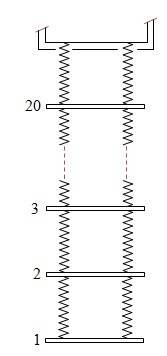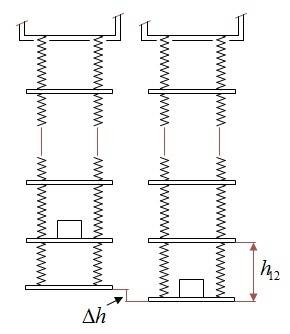# Springs in a row

• Karol
The system looses energy when the step rises...That's correct. In summary, this conversation discusses a problem involving a princess throwing a ladder of 20 plates of thickness d and mass m each, connected with springs of constant k and loose length l0, to rescue her lover who is at a height H. The conversation discusses the elongation of the springs, the total length of the ladder, the change in the ladder's length when the lover climbs to different steps, the change in the system's mechanical energy when the lover climbs from step 1 to 2, the ladder's length when the lover sits on the n-th step, and the length of the lover's way if he climbs up slowly to the window. The

## Homework Statement[/B] In a tower a princess throws a ladder of 20 plates of thickness d and mass m each, which are connected with springs of constant k and loose length l0. the window is at height H.
1) What's the elongation of each of the spring pairs?
2) What's the total ladder's length.
3) The princess's lover, mass M, climbs to the first step and waits to the oscillations to stop. what is the ladder's length now.
4) The lover now climbs to the second step and waits. what's the length now.
5) When he passes from step 1 to 2, what's the change in the system's mechanic energy?
6) When he sits on the n-th step, what's the ladder's length?
7) If he would climb up slowly to the window, what would be the length of his way?

## Homework Equations

Equivalent spring of two springs in a row: ##\frac{1}{k_{eq}}=\frac{1}{k_1}+\frac{1}{k_2}##
Equivalent spring of two parallel springs: ##k_{eq}=k_1+k_2##
$$\sum_1^n n=\frac{n(n+1)}{2}$$

## The Attempt at a Solution

1) The elongation of each of the n-th pair of strings: ##nmg=\overline k\cdot \Delta x_n~\rightarrow~\Delta x_n=\frac{nmg}{2k}##
2) The total length of n stages of the ladder, starting from the bottom:
$$L_n=n(l_0+d)+\sum \Delta x_n=n(l_0+d)+\frac{mg}{2k}\sum n=n\left[ l_0+d+\frac{mg(n+1)}{4k}\right]$$
Length of 20 steps:
$$L_{20}=20\left[ l_0+d+\frac{21mg}{4k} \right]$$
The distance of the n-th step from the window (considering only the step's mass m), ##L_{(20-n)m}##, is the reduction of the n stage's length, measured from the bottom, from the total length:
$$L_{(20-n)m}=\left( 20(l_0+d)+\sum_1^{20} \Delta x_n \right)-\left(n(l_0+d)+\sum_1^n \Delta x_n \right)=(20-n)(l_0+d)+205-\frac{n(n+1)}{2}$$
3) The equivalent spring constant of n pairs of parallel springs in a row is ##k_{eq}=\frac{2k}{n}##
The equivalent spring constant of the springs above the n-th step is ##k_{eq}=\frac{2k}{21-n}##
When M is on the first step:
$$L_{20m+M}=20\left[ l_0+d+\frac{21mg}{4k} \right]+\frac{10Mg}{k}$$
When M is on the n-th step it's distance from the window, ##L_{(20-n)M}##, is:
$$L_{(20-n)M}=L_{(20-N)m}+L_{nM}=(20-n) (l_0+d)+205-\frac{n(n+1)}{2}+\frac{Mg(21-n)}{2k}$$5) When M climbs to the second step the total energy of the ladder and M system increases by Mg x (difference of height between the steps). the first step rises a bit as M leaves it but it's energy gain is balanced by the energy loss of the spring's contraction.
$$h_{12}=L_{20+M}-L_{19+M}=20\left[ l_0+d+\frac{21mg}{4k} \right]+\frac{20Mg}{2k}-19\left[ l_0+d+\frac{5mg}{k} \right]-\frac{19Mg}{2k}=l_0+d+\left( 10m+\frac{M}{2} \right)\frac{g}{k}$$
$$\Delta h=\frac{Mg}{2k}$$
The energy gain:
$$\Delta E=Mg\cdot h_{12}$$
6) It doesn't matter on which step M sits, the expansion of the springs above it is the same as if he were sitting on the first one since the force is transmitted to all the steps. i mean, if he sits on the first step and i measure the elongation in the n-th set of springs, the same elongation would be if he sat on that n-th step.
So, the distance he crosses on his way up is just the total length of the ladder with him on the first step:

There are problems with the solution as well as statement of the problem.
Statement: How does the prince throw the ladder holding the 20th plate from the top or holding the first plate from below.

Solution; The equivalence formula of spring works when there is no connecting mass between the springs.
Although the background of eh problem is quite fairy, you will have to labor out the solution.

Let'sthink said:
Statement: How does the prince throw the ladder holding the 20th plate from the top or holding the first plate from below.
The princess throws the ladder from the top. She does not hold any plate. As the diagram shows, the highest plate is one spring length below the window.

Let'sthink said:
Solution; The equivalence formula of spring works when there is no connecting mass between the springs.
I don't see why the intervening masses would invalidate it. The force/extension relationship is linear. An additional force Mg on each drop will add extension Mg/(2k) to the length of each drop.

Karol said:
When M is on the n-th step
Careful here. When on the first step (n=1?) there are 20 drops feeling his weight, not 20-1.

Karol said:
first step rises a bit as M leaves it but it's energy gain is balanced by the energy loss of the spring's contraction.
I think you will find that does not cancel out.

I agree with your answer to 6, but in 7 I'm not sure how you are supposed to treat the step in which he mounts the very first step. The ladder will elongate as he does so.

haruspex said:
Careful here. When on the first step (n=1?) there are 20 drops feeling his weight, not 20-1.
When M is on the n-th step it's distance from the window, ##L_{[20 \rightarrow (n-1)]M}##, is:
$$L_{[20 \rightarrow (n-1)]M}=L_{[20 \rightarrow (n-1)]m}+L_{nM}=\left( 20(l_0+d)+\sum_1^{20} \Delta x_n \right)-\left( (n-1)(l_0+d)+\sum_1^{n-1} \Delta x_n \right)=20\left[ l_0+d+\frac{21mg}{4k} \right]-(n-1)\left[ l_0+d+\sum_1^{n-1}\Delta x_n \right]+\frac{10Mg}{k}=(19-n)(l_0+d)+\frac{105mg}{k}-\frac{n^2-n}{2}+\frac{10Mg}{k}$$
About the rest i will continue tomorrow morning

haruspex said:
I think you will find that does not cancel out.
The energy with M:
$$(M+m)g=2k\cdot x~\rightarrow~x=\frac{(M+m)g}{2k},~~E_1=\frac{1}{2}\cdot 2k \cdot x^2=...=\frac{(M+m)^2g^2}{4k}$$
The energy without M:
$$E_2=\frac{m^2g^2}{4k}$$
The difference in energy:
$$\Delta E_{spring}=E_1-E_2=\frac{Mg^2}{4k}(M+2m)$$
What's the height's energy gain by the rising of the step as M leaves:
The difference in height as M leaves is:
$$\Delta h=\frac{Mg}{2k},~~\Delta E_m=mg\cdot \Delta h=\frac{Mmg^2}{2k}$$
As M leaves the system gains more energy than than it looses by the contraction of the springs:
$$\Delta E=Mg\cdot h_{12}+\Delta E_m-\Delta E_{spring}$$
$$\Delta E=Mg\left[ l_0+d+\left( 10m+\frac{M}{2} \right) \frac{g}{k} \right]+\frac{Mmg^2}{2k}-\frac{Mg^2}{4k}(M+2m)>0$$
How can it be?

Karol said:
How can it be?
What puzzles you? There is some cancellation available in your last two terms above. That done, you see the increase in the energy of the system is the prince's gain in PE less a bit for the relaxation and rise of the bottom link. This comes from the work done by the prince in stepping up.

Thank you Haruspex (the prince...)

I forgot question 6, the total way M moves from the first step to the window, assuming he is at rest on the first. is it correct:
Karol said:
6) It doesn't matter on which step M sits, the expansion of the springs above it is the same as if he were sitting on the first one since the force is transmitted to all the steps. i mean, if he sits on the first step and i measure the elongation in the n-th set of springs, the same elongation would be if he sat on that n-th step.
So, the distance he crosses on his way up is just the total length of the ladder with him on the first step:

Karol said:
I forgot question 6, the total way M moves from the first step to the window, assuming he is at rest on the first. is it correct:
Yes, I agreed with that (post #6).

Thank you Haruspex very much

I am wrong again, i meant question 7 instead of 6. the total distance M will climb is the stretched ladder length as he sits on the first step:
$$L_{20m+M}=20\left[ l_0+d+\frac{21mg}{4k} \right]+\frac{10Mg}{k}$$

Karol said:
I am wrong again, i meant question 7 instead of 6. the total distance M will climb is the stretched ladder length as he sits on the first step:
$$L_{20m+M}=20\left[ l_0+d+\frac{21mg}{4k} \right]+\frac{10Mg}{k}$$
As I wrote in post #6, I agree with that too, on the assumption that it means the distance climbed after (3). I.e. It does not include getting onto the first step.

Right, thank you for your patience Haruspex